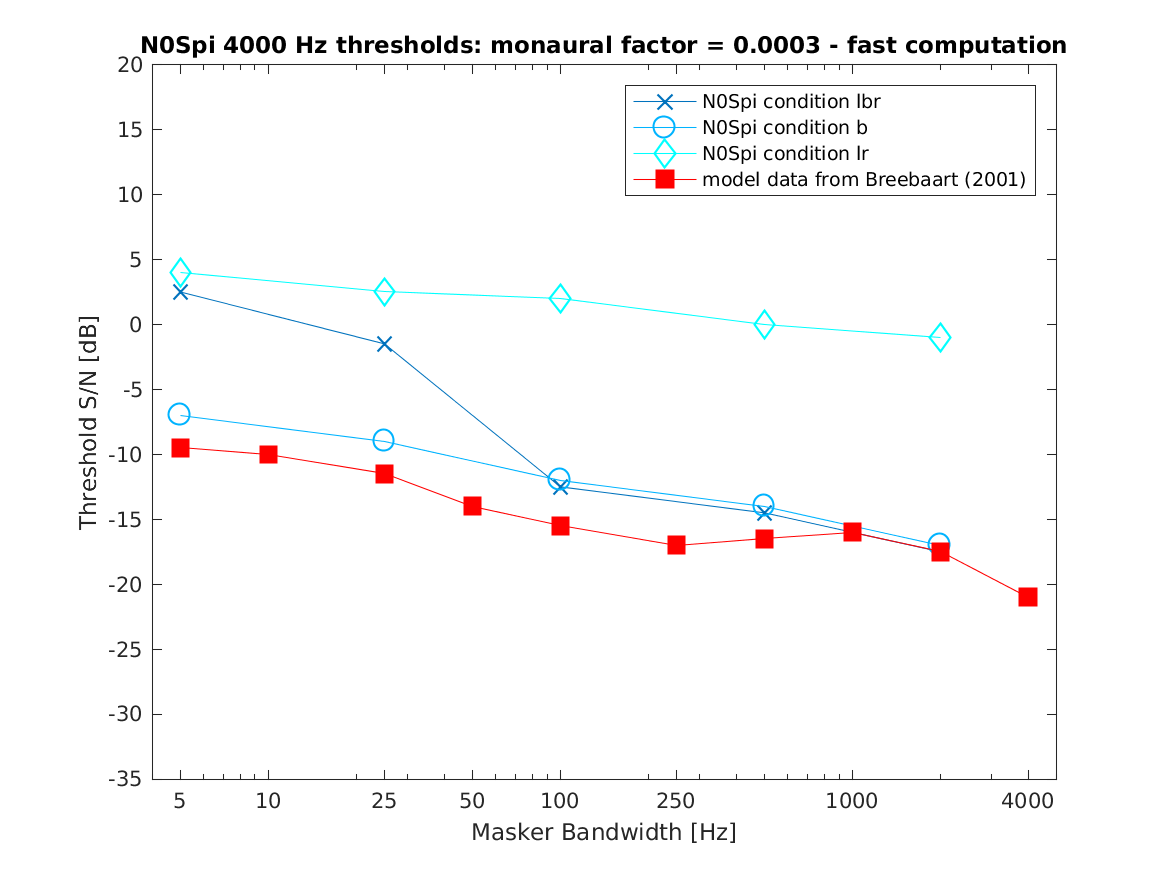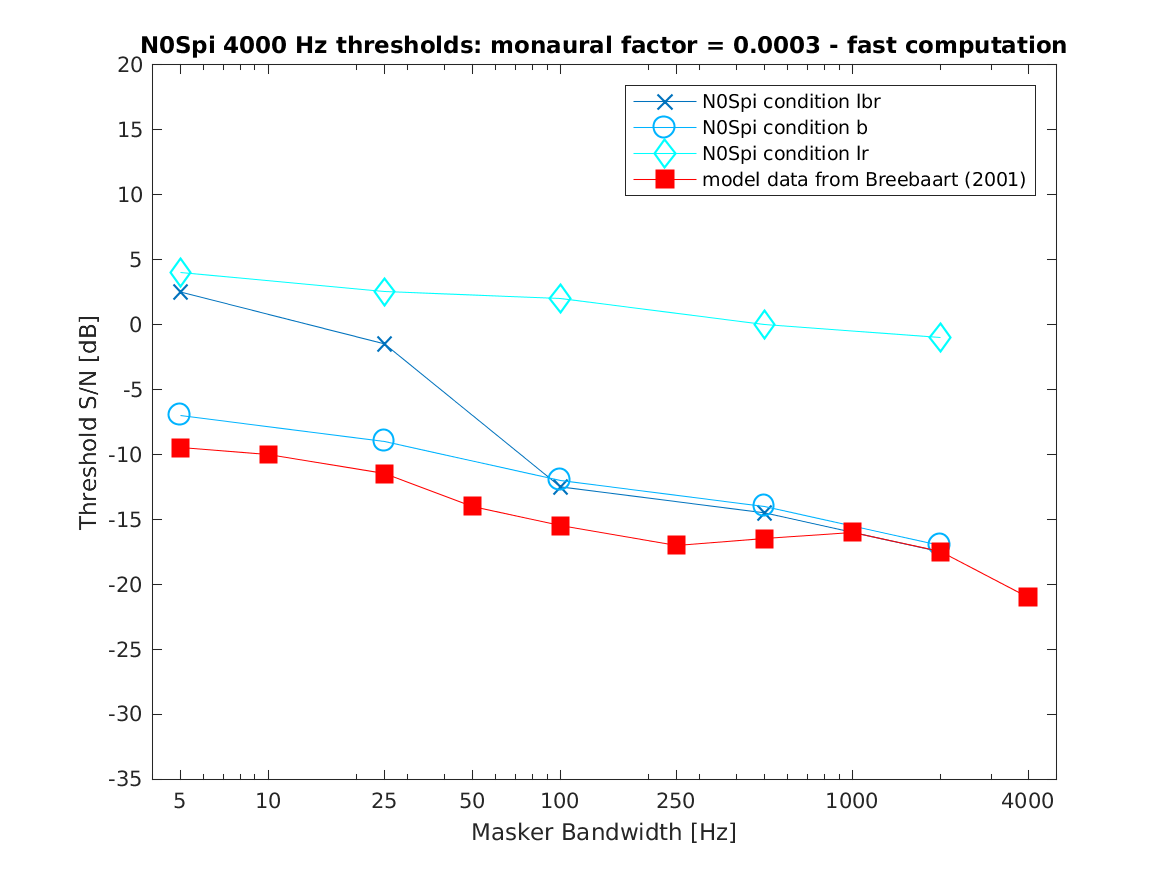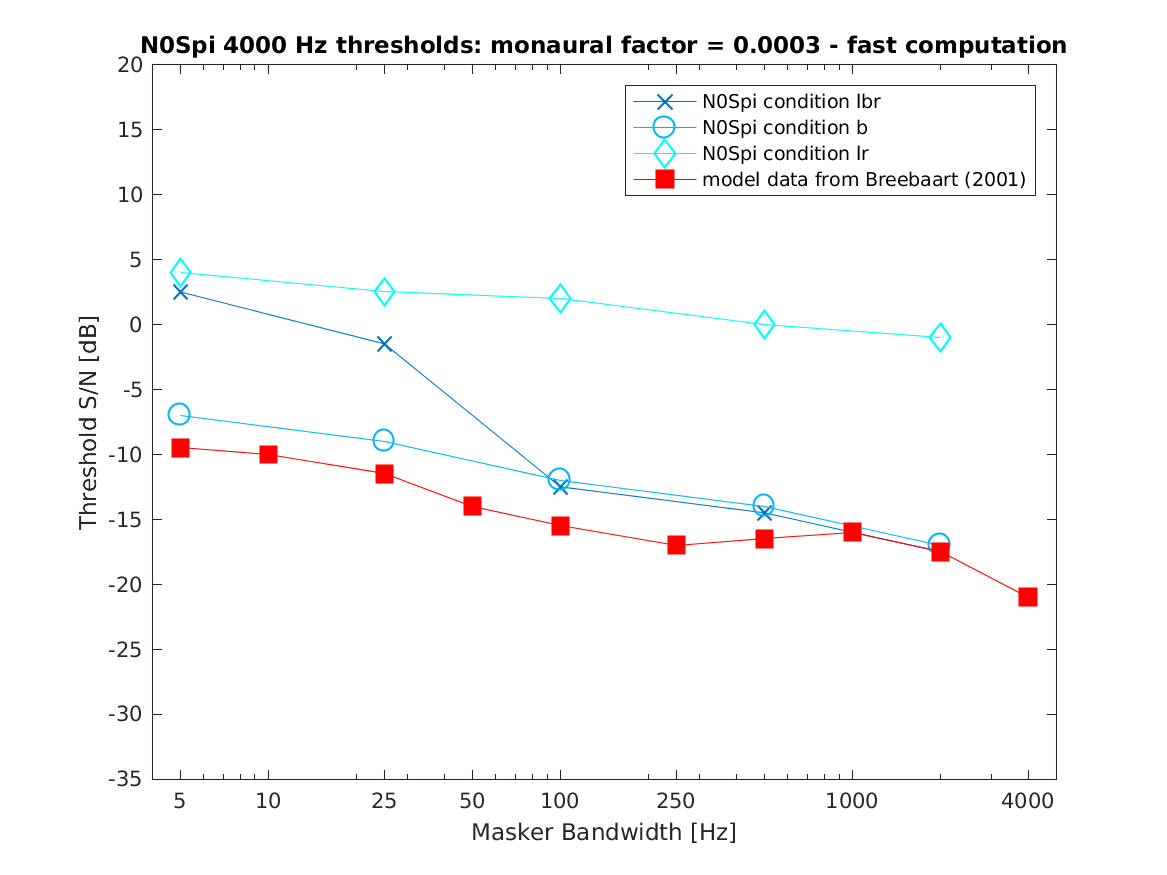# THE AUDITORY MODELING TOOLBOX

Applies to version: 1.0.0

Go to function

# DEMO_BREEBAART2001 - Demo for binaural processingmodel from Breebaart et al. (2001)

## Description

demo_breebaart2001(flags) demonstrates how to compute N0S0, N0Spi and NpiS0 thresholds for different combinations of the monaural and binaural central processor for a center frequency of 1000 or 4000 Hz using the model from Breebaart et al. (2014).

The N0Spi condition is default. The following combinations for 4000 Hz are calculated:

lbr Compute decision from combination of left, binaural and
right result. If the binaural result is zero, don't use it. In this condition lbr is equal to lBr, because the binaural result is not zero.

b Compute decision from binaural result only.

lr Compute decision from left and right result.

Set flag to the following flags to shows other conditions:

 'N0S0' N0S0 thresholds for differnt combinations of the monaural and binaural processor for a center frequency of 4000 Hz. The following combinations are calculated: lbr Compute decision from combination of left, binaural and right result. If the binaural result is zero, don't use it. In this condition lbr is equal to lr. lBr Compute decision from combination of left, binaural and right result even if the binaural result is zero. 'NpiS0' NpiS0 thresholds for differnt combinations of the monaural and binaural processor for a center frequency of 1000 Hz. The following combinations are calculated: 'lbr' Compute decision from combination of left, binaural and right result. If the binaural result is zero, don't use it. In this condition lbr is equal to lBr, because the binaural result is not zero. 'b' Compute decision from binaural result only. 'lr' Compute decision from left and right result. 'exact' The threshold is computed as a mean value of 6 repetitions per bandwidth. To get results faster the computational results are stored in cache and can be downloaded. 'fast' The threshold as the result of one experimental run per bandwidth. The number of bandwidth values is half the number of values at the 'exact' computation. The fast computation is default.

demo_breebaart2001('N0Spi') shows

demo_breebaart2001('N0Spi');N0Spi thresholds as a function of the masker bandwidth for a center frequency of 4000 Hz and lbr, b and lr conditions in comparison with the model results of Breebaart et al. (2001b).

demo_breebaart2001('N0S0') shows

demo_breebaart2001('N0S0');N0S0 thresholds as a function of the masker bandwidth for a center frequency of 4000 Hz and lbr and lBr conditions in comparison with experimental results of van de Par and Kohlrausch (1999).

demo_breebaart2001('NpiS0') shows

demo_breebaart2001('NpiS0');NpiS0 thresholds as a function of the masker bandwidth for a center frequency of 4000 Hz and lbr, b and lr conditions in comparison with the model results of Breebaart et al. (2001b).

## References:

J. Breebaart, S. van de Par, and A. Kohlrausch. Binaural processing model based on contralateral inhibition. II. Dependence on spectral parameters. J. Acoust. Soc. Am., 110:1089--1104, August 2001.

S. van de Par and A. Kohlrausch. Dependence of binaural masking level differences on center frequency, masker bandwidth, and interaural parameters. J. Acoust. Soc. Am., 106(4):1940--1947, 1999.